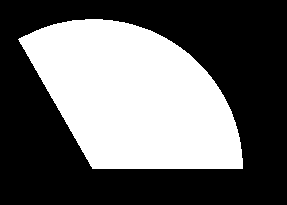# pieslice() function in C

pieslice() draws and fills a pie slice with center at (x, y) and given radius r. The slice travels from s_angle to e_angle which are starting and ending angles for the pie slice. The angles for pie-slice are given in degrees and are measured counterclockwise.

Syntax :

```void pieslice(int x, int y, int s_angle,
int e_angle, int r);

where,
(x, y) is center of the circle.
r is the radius of the circle.
s_angle and e_angle are the starting
and ending angles respectively.
```

Examples :

```Input : x = 300, y = 300, s_angle = 0 ,
e_angle = 120, r = 150
Output :Input : x = 300, y = 300, s_angle = 30 ,
e_angle = 100, r = 200
Output :```

Below is the implementation for the pieslice() function:

 `// C Implementation for drawing pieslice ` `#include ` ` `  `// driver code ` `int` `main() ` `{ ` `    ``// gm is Graphics mode which  ` `    ``// is a computer display mode  ` `    ``// that generates image using pixels. ` `    ``// DETECT is a macro defined in ` `    ``// "graphics.h" header file ` `    ``int` `gd = DETECT, gm; ` ` `  `    ``// initgraph initializes the  ` `    ``// graphics system by loading a  ` `    ``// graphics driver from disk ` `    ``initgraph(&gd, &gm, ``""``); ` ` `  `    ``// pieslice function ` `    ``pieslice(300, 300, 0, 120, 150); ` ` `  `    ``getch(); ` ` `  `    ``// closegraph function closes the  ` `    ``// graphics mode and deallocates all  ` `    ``// memory allocated by graphics system . ` `    ``closegraph(); ` ` `  `    ``return` `0; ` `} `

Output :

``````

My Personal Notes arrow_drop_upCheck out this Author's contributed articles.

If you like GeeksforGeeks and would like to contribute, you can also write an article using contribute.geeksforgeeks.org or mail your article to contribute@geeksforgeeks.org. See your article appearing on the GeeksforGeeks main page and help other Geeks.

Please Improve this article if you find anything incorrect by clicking on the "Improve Article" button below.

Improved By : Akanksha_Rai

Article Tags :
Practice Tags :

1

Please write to us at contribute@geeksforgeeks.org to report any issue with the above content.